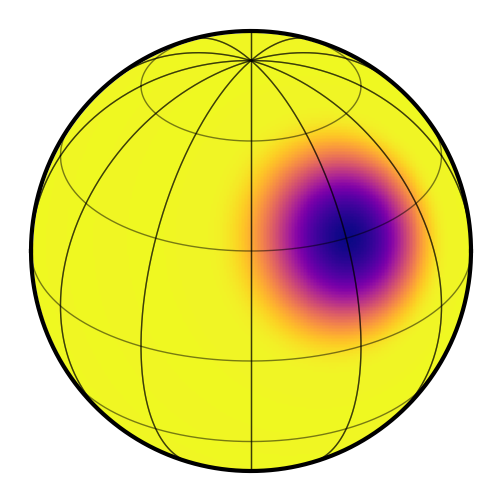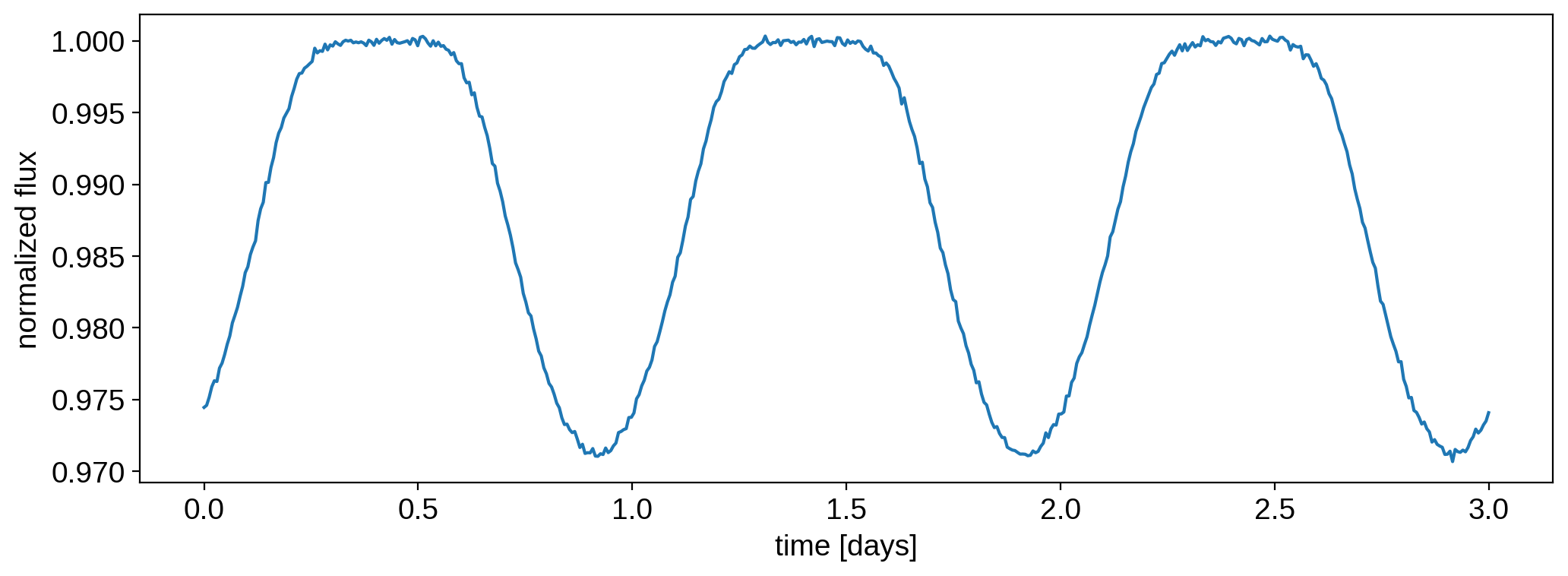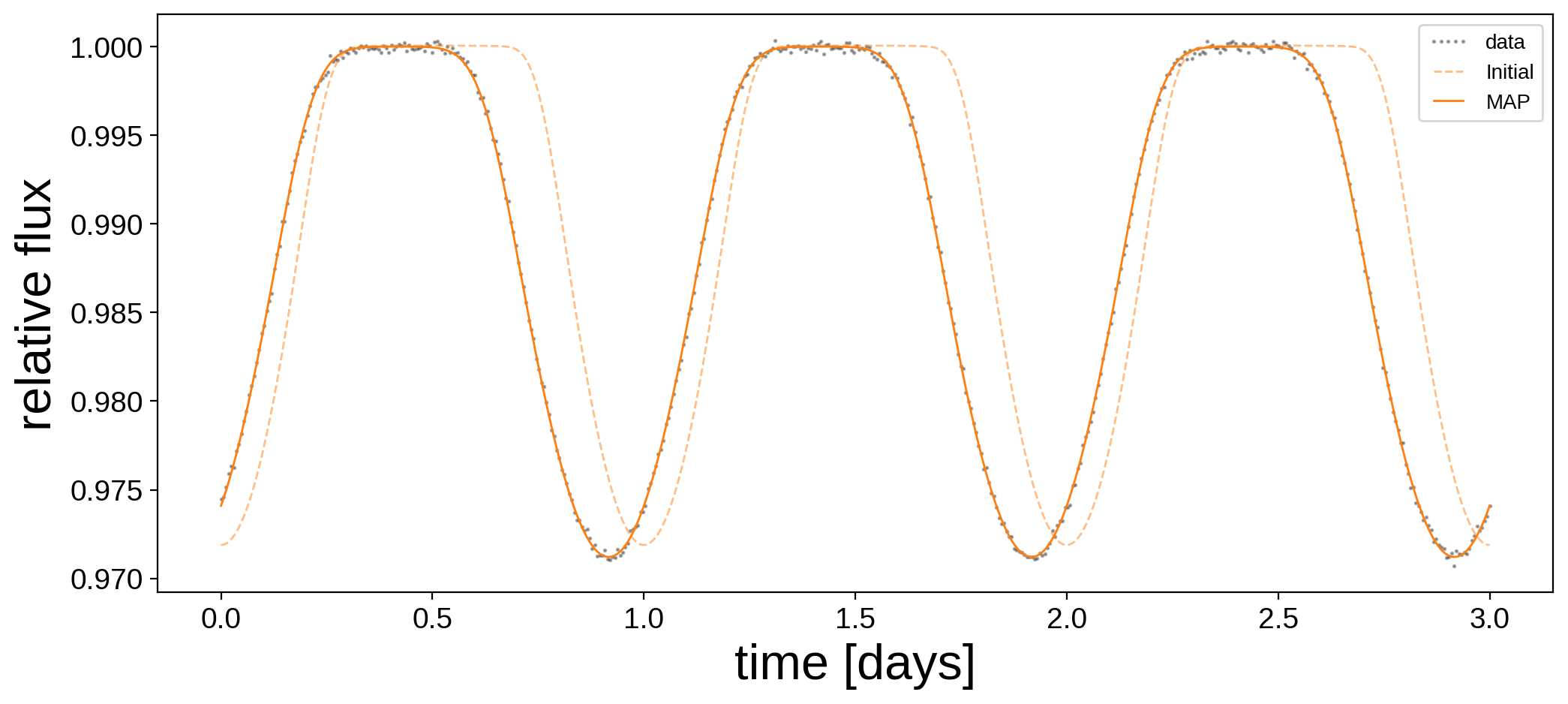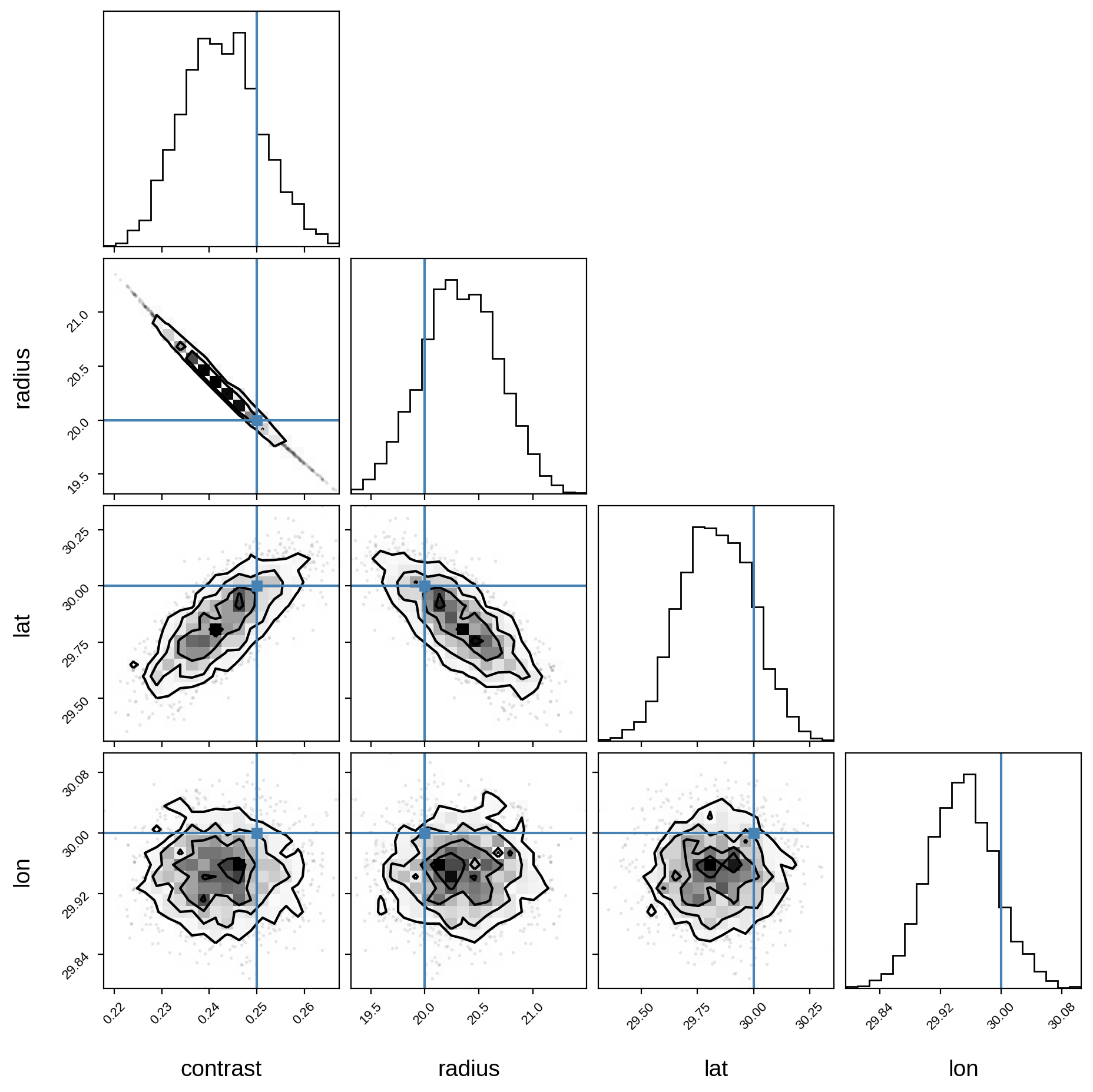Note

This tutorial was generated from a Jupyter notebook that can be downloaded here.

# Where’s the spot?¶

An example of how to solve for the location, amplitude, and size of a star spot.

As we discuss in this notebook, starry isn’t really meant for modeling discrete features such as star spots; rather, starry employs spherical harmonics to model the surface brightness distribution as a smooth, continuous function. We generally recommend approaching the mapping problem in this fashion; see the Eclipsing Binary tutorials for more information on how to do this. However, if you really want to model the surface of a star with star spots, read on!

Let’s begin by importing stuff as usual:

:

import numpy as np
import starry
import exoplanet as xo
import pymc3 as pm
import pymc3_ext as pmx
import matplotlib.pyplot as plt
from corner import corner

starry.config.quiet = True


We discussed how to add star spots to a starry map in this tutorial. Here, we’ll generate a synthetic light curve from a star with a single large spot with the following parameters:

:

# True values
truth = dict(contrast=0.25, radius=20, lat=30, lon=30)


Those are, respectively, the fractional amplitude of the spot, its standard deviation (recall that the spot is modeled as a Gaussian in $$\cos\Delta\theta$$, its latitude and its longitude.

To make things simple, we’ll assume we know the inclination and period of the star exactly:

:

# Things we'll assume are known
inc = 60.0
P = 1.0


Let’s instantiate a 15th degree map and give it those properties:

:

map = starry.Map(15)
map.inc = inc
map.spot(
contrast=truth["contrast"],
lat=truth["lat"],
lon=truth["lon"],
)
map.show()Now we’ll generate a synthetic light curve with some noise:

:

t = np.linspace(0, 3.0, 500)
flux0 = map.flux(theta=360.0 / P * t).eval()
np.random.seed(0)
flux_err = 2e-4
flux = flux0 + flux_err * np.random.randn(len(t))


Here’s what that looks like:

:

plt.plot(t, flux)
plt.xlabel("time [days]")
plt.ylabel("normalized flux");In this notebook, we are going to derive posterior constraints on the spot properties. Let’s define a pymc3 model and within it, our priors and flux model:

:

with pm.Model() as model:

# Priors
contrast = pm.Uniform("contrast", lower=0.0, upper=1.0, testval=0.5)
lat = pm.Uniform("lat", lower=-90.0, upper=90.0, testval=0.1)
lon = pm.Uniform("lon", lower=-180.0, upper=180.0, testval=0.1)

# Instantiate the map and add the spot
map = starry.Map(ydeg=15)
map.inc = inc

# Compute the flux model
flux_model = map.flux(theta=360.0 / P * t)
pm.Deterministic("flux_model", flux_model)

# Save our initial guess
flux_model_guess = pmx.eval_in_model(flux_model)

# The likelihood function assuming known Gaussian uncertainty
pm.Normal("obs", mu=flux_model, sd=flux_err, observed=flux)


Note

It’s important to define a nonzero testval for both the latitude and longitude of the spot. If you don’t do that, it’s likely the optimizer will get stuck at lat, lon = 0, 0, which is a local maximum of the likelihood.

We’ve placed some generous uniform priors on the four quantities we’re solving for. Let’s run a quick gradient descent to get a good starting position for the sampler:

:

with model:
map_soln = pmx.optimize(start=model.test_point)

optimizing logp for variables: [lon, lat, radius, contrast]

100.00% [64/64 00:00<00:00 logp = 3.545e+03]


message: Optimization terminated successfully.
logp: -278193.2524187515 -> 3545.1103694957396


Here’s the data and our initial guess before and after the optimization:

:

plt.figure(figsize=(12, 5))
plt.plot(t, flux, "k.", alpha=0.3, ms=2, label="data")
plt.plot(t, flux_model_guess, "C1--", lw=1, alpha=0.5, label="Initial")
plt.plot(
t, pmx.eval_in_model(flux_model, map_soln, model=model), "C1-", label="MAP", lw=1
)
plt.legend(fontsize=10, numpoints=5)
plt.xlabel("time [days]", fontsize=24)
plt.ylabel("relative flux", fontsize=24);And here are the maximum a posteriori (MAP) values next to the true values:

:

print("{0:12s} {1:10s} {2:10s}".format("", "truth", "map_soln"))
for key in truth.keys():
print("{0:10s} {1:10.5f} {2:10.5f}".format(key, truth[key], map_soln[key]))

             truth      map_soln
contrast      0.25000    0.24234
lat          30.00000   29.83735
lon          30.00000   29.94841


Not bad! Looks like we recovered the correct spot properties. But we’re not done! Let’s get posterior constraints on them by sampling with pymc3. Since this is such a simple problem, the following cell should run in about a minute:

:

with model:
trace = pmx.sample(tune=250, draws=500, start=map_soln, chains=4, target_accept=0.9)

Sequential sampling (4 chains in 1 job)

100.00% [750/750 00:37<00:00 Sampling chain 0, 0 divergences]
100.00% [750/750 00:38<00:00 Sampling chain 1, 0 divergences]
100.00% [750/750 00:36<00:00 Sampling chain 2, 0 divergences]
100.00% [750/750 00:33<00:00 Sampling chain 3, 0 divergences]
Sampling 4 chains for 250 tune and 500 draw iterations (1_000 + 2_000 draws total) took 146 seconds.


Plot some diagnostics to assess convergence:

:

var_names = ["contrast", "radius", "lat", "lon"]
display(pm.summary(trace, var_names=var_names))

mean sd hdi_3% hdi_97% mcse_mean mcse_sd ess_mean ess_sd ess_bulk ess_tail r_hat
contrast 0.243 0.008 0.228 0.258 0.000 0.000 677.0 677.0 664.0 612.0 1.0
radius 20.326 0.354 19.667 20.987 0.014 0.010 677.0 677.0 666.0 608.0 1.0
lat 29.834 0.158 29.566 30.144 0.005 0.004 1017.0 1017.0 1013.0 1220.0 1.0
lon 29.948 0.044 29.869 30.035 0.001 0.001 1608.0 1608.0 1609.0 1446.0 1.0

And finally, the corner plot showing the joint posteriors and the true values (in blue):

:

samples = pm.trace_to_dataframe(trace, varnames=var_names)
corner(samples, truths=[truth[name] for name in var_names]);

WARNING:root:Pandas support in corner is deprecated; use ArviZ directlyWe’re done! It’s easy to extend this to multiple spots, simply by calling map.spot once for each spot in the model, making sure you define new pymc3 variables for the spot properties of each spot.